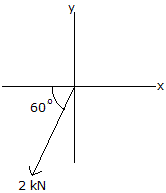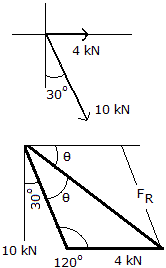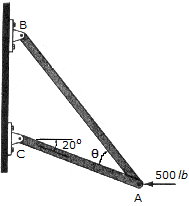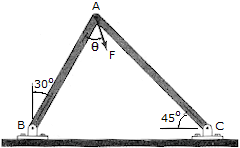# Engineering Mechanics - Force Vectors

### Exercise :: Force Vectors - General Questions

• Force Vectors - General Questions
21.Determine the magnitude of the x and y components of the 2—kN force.

 A. Fx = -1.414 kN, Fy = -1.414 kN B. Fx = -1.000 kN, Fy = -1.732 kN C. Fx = -1.732 kN, Fy = -1.000 kN D. Fx = -4.000 kN, Fy = -2.312 kN

Explanation:

No answer description available for this question. Let us discuss.

22.Cable BC exerts a force of F = 28 N on the top of the flagpole. Determine the projection of this force along the positive z axis of the pole.

 A. F = 24 N B. F = -24 N C. F = 12 N D. F = 12 N

Explanation:

No answer description available for this question. Let us discuss.

23.Determine the magnitudes of the resultant force and its direction measured from the positive x axis.

 A. R = 12.49 kN,= 43.9° CW B. R = 13.6 kN,= 21.5° CW C. R = 14.00 kN,= 60.0° CW D. R = 10.80 kN,= 68.2° CW

Explanation:

No answer description available for this question. Let us discuss.

24.Determine the design angle(<90°) bretween the two struts so that the 500-lb horizontal force has a component of 600lb directed from A toward C. That is the component of force acting along member AB?

 A. FAB = 321 lb,= 40.0° B. FAB = 383 lb,= 36.9° C. FAB = 171.1 lb,= 20.0° D. FAB = 215 lb,= 52.7°

Explanation:

No answer description available for this question. Let us discuss.

25.Determine the magnitude and directionof F so that this force has components of 40 lb acting from A toward B and 60lb acting from A toward C on the frame.

 A. F = 44.7 lb,= 22.9° B. F = 80.3 lb,= 46.2° C. F = 62.9 lb,= 37.1° D. F = 72.1 lb,= 56.3°